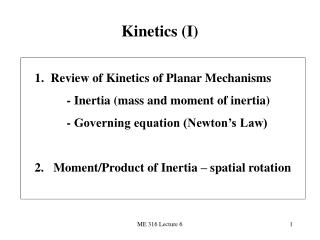Download PresentationKinetics (I)

# Kinetics (I)

Download Presentation## Kinetics (I)

- - - - - - - - - - - - - - - - - - - - - - - - - - - E N D - - - - - - - - - - - - - - - - - - - - - - - - - - -
##### Presentation Transcript

1. Kinetics (I) • Review of Kinetics of Planar Mechanisms • - Inertia (mass and moment of inertia) • - Governing equation (Newton’s Law) • 2. Moment/Product of Inertia – spatial rotation ME 316 Lecture 6

2. Review of Planar Kinetics of a Rigid Body Kinetics: how does a body move under the force or moment ? If there is no any force applied to a body then the body will remain its current status Motion or no-motion in a specific direction Total resultant force in a direction The above is called “Newton First Law” ME 316 Lecture 6

3. Review of Planar Kinetics of a Rigid Body The basic evidence to support the Newton First Law is the inertia • A property of an object; • A measure of how difficult or easy the motion of a body can be changed; • 3. An inherent resistance to change ME 316 Lecture 6

4. Review of Planar Kinetics of a Rigid Body Two types of inertia depending on types of causes Cause is force: inertia is mass (m) Cause is moment: inertia is the moment of inertia (IP) A IA≠ IB B ME 316 Lecture 6

5. B A Review of Planar Kinetics of a Rigid Body Example (to be filled) IA≠ IB ME 316 Lecture 6

6. B G Review of Planar Kinetics of a Rigid Body Parallel – axis theorem (to be filled) G is the center of gravity ME 316 Lecture 6

7. Review of Planar Kinetics of a Rigid Body Kinetic energy (K) • Translation (T) (to be filled) • Rotation (R) (to be filled) • General (T + R) (to be filled) ME 316 Lecture 6

8. Review of Planar Kinetics of a Rigid Body Kinetics Equation Translation: X and Y axes may not be horizontal or vertical; rather they could be in any direction but not in parallel. ME 316 Lecture 6

9. Review of Planar Kinetics of a Rigid Body Rotation: Case 1: G P May not be fixed ME 316 Lecture 6

10. Review of Planar Kinetics of a Rigid Body Case 2: P=G (mass center) ME 316 Lecture 6

11. Review of Planar Kinetics of a Rigid Body Case 3: when P is fixed point ME 316 Lecture 6

12. Review of Planar Kinetics of a Rigid Body Translation and rotation or or ME 316 Lecture 6

13. z Moment/product of Inertia of Spatial Rotation ME 316 Lecture 6

14. Product of Inertia Orthogonal planes ME 316 Lecture 6

15. Product of Inertia x ME 316 Lecture 6

16. Product of Inertia If either one or both of the orthogonal planes are planes of symmetry for the mass, the product of inertia with respect to these planes will be zero. ME 316 Lecture 6

17. Moment /Product of Inertia Parallel-axis and parallel-plane theorems ME 316 Lecture 6

18. Moment /Product of Inertia Inertia Tensor – a compact way to express ME 316 Lecture 6

19. Moment /Product of Inertia Principal axes - principal moments of inertia If the coordinate axes are oriented such that two of the three orthogonal planes containing the axes are planes of symmetry for the body, then all the products of inertia for the body are zero with respect to the coordinate planes, and hence the coordinate axes are principal axes of inertia ME 316 Lecture 6

20. Moment /Product of Inertia Moment of inertia about an arbitrary axis ME 316 Lecture 6

21. Moment /Product of Inertia The bent rod ABCD has a weight of 1.5 lb/ft Example 1 Find: the location of center of gravity G and Ix’, Iy’, Iz’ ME 316 Lecture 6

22. Moment /Product of Inertia The 1.5 Kg rod and 4 Kg disk Example 2 Find: Iz’ of the composite body ME 316 Lecture 6

23. Moment /Product of Inertia The bent rod OABC has mass of 4 Kg/m Example 3 Find: Ix’x’ of the rod ME 316 Lecture 6